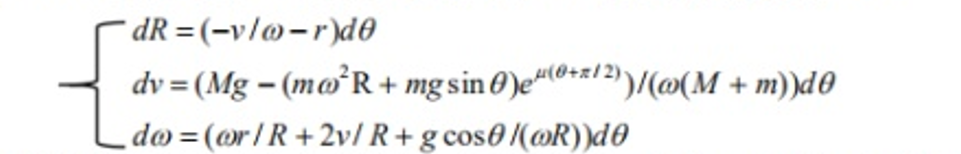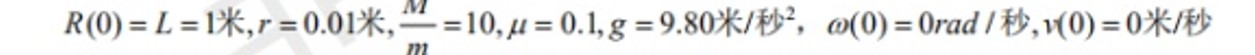Matlab求常微分方程组的解析解

21 篇文章 4 订阅

（强烈建议大家有问题多看官方文档，非常有用）

1. 一阶常微分方程求解（无初值）

syms y(t) a; %syms 定义方程用的变量和未知常量(可有可无)。y(t)表示y是关于t的函数，求解t。
eqn=diff(y,t)==a*y; %diff(y,t)即dy/dt，默认为1阶。
S=dsolve(eqn) %dsolve()为求解函数，将方程放入即可

S =
C2*exp(a*t)

2. 二阶常微分方程求解（无初值）

syms y(t) a; %syms 定义方程用的变量和未知常量。y(t)表示y是关于t的函数，求解t。
eqn=diff(y,t,2)==a*y; %diff(y,t,2)即dy²/dt²，第三个参数表示阶数，更高阶数一样。
S=dsolve(eqn) %dsolve()为求解函数，将方程放入即可

S =
C3*exp(a^(1/2)*t) + C4*exp(-a^(1/2)*t)

3. 二阶微分方程求解（有初值）

syms y(t) a; %syms 定义方程用的变量和未知常量。y(t)表示y是关于t的函数，求解t。
eqn=diff(y,t,2)==a*y; %定义diff(y,t,2)即dy²/dt²，第三个参数表示阶数。
Dy=diff(y,t); %定义方程Dy为dy/dt；
cond=[y(0)==5,Dy(0)==1]; %定义初值，t=0时，y=5,dy/dt=1;
S=dsolve(eqn,cond) %dsolve()为求解函数，将方程放入即可

S =
(exp(a^(1/2)*t)*(5*a^(1/2) + 1))/(2*a^(1/2)) + (exp(-a^(1/2)*t)*(5*a^(1/2) - 1))/(2*a^(1/2))

4. 一阶微分方程组求解（有初值）

dz/dt=-y

syms y(t) z(t); %syms 定义方程用的变量
eqn=[diff(y,t)==z,diff(z,t)==-y]; %定义方程组dy/dt=z，dz/dt=-y
cond=[y(0)==1,z(1)==1]; %定义初值，t=0时，y=5；t=1时，y=1；
[ySol zSol]=dsolve(eqn,cond) %dsolve()为求解函数，将方程放入即可

ySol =
cos(t - 1)/(2*cos(1)) - cos(t + 1)/(2*cos(1)) + cos(t - atan(1/cos(1)))*(1/cos(1)^2 + 1)^(1/2)
zSol =
sin(t + 1)/(2*cos(1)) - sin(t - 1)/(2*cos(1)) + (cos(t + atan(cos(1)))*(cos(1)^2 + 1)^(1/2))/cos(1)

5. 无法求解情况

syms y(t) %syms 定义方程用的变量和常量
eqn=diff(y,2)==(1-y^2)*diff(y)-y; %定义方程dy²/dt²=(1-y²)*dy/dt-y
cond=y(0)==1; %定义初值，t=0时，y=1
S=dsolve(eqn,cond) %dsolve()为求解函数，将方程放入即可

> In dsolve (line 201)
S =
[ empty sym ]

6. 无法求解解决方案syms R(t) v(t) w(t);
eqns=[diff(R,t)==-v/w-0.01,...
diff(v,t)==(10*9.8-(w^2*R+9.8*sin(t))*exp(0.1*(t+pi/2)))/(w*11),...
diff(w,t)==w*0.01/R+2*v/R+9.8*cos(t)/(w*R)];
cond=[R(0)==1,v(0)==0,w(0)==1e3];
S=dsolve(eqns,cond);

7. 加油！

09-127万+

10-15
03-22400
01-2219万+
02-088222
08-106881
12-133万+
09-096186
03-16150
09-255668
08-091万+
11-28529
02-094351
03-17703
05-2913万+
04-304万+
04-301万+
03-091176y=520（2sinM+sin2M）

¥2 ¥4 ¥6 ¥10 ¥20余额支付 (余额：-- )扫码支付获取中扫码支付点击重新获取扫码支付1.余额是钱包充值的虚拟货币，按照1:1的比例进行支付金额的抵扣。
2.余额无法直接购买下载，可以购买VIP、C币套餐、付费专栏及课程。余额充值